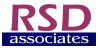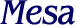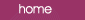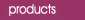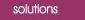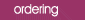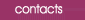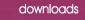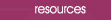Normalised Individuals Charts The values plotted on a Normalised Individuals Chart are obtained by subtracting the grand average of all of the readings from each individual value.  The values are then plotted around a zero reference line.  Two formats of Normalised Individuals Charts are described in the AIAG manual and the examples using the AIAG data are shown below: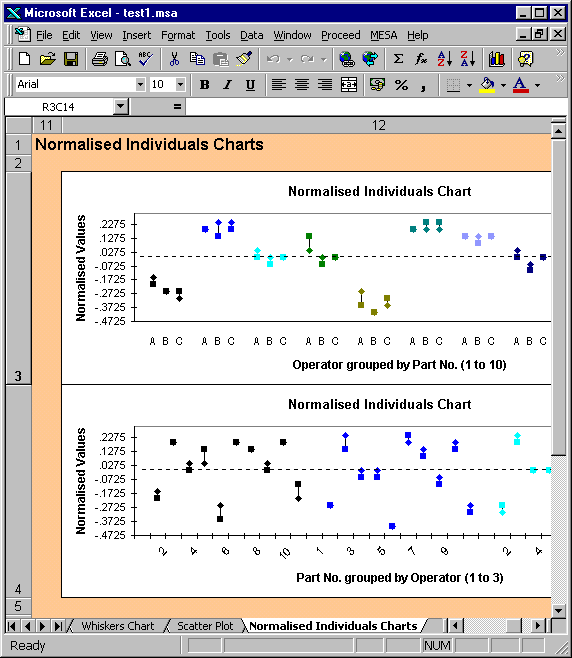In the upper plot the normalised values have been grouped by part number, e.g. all of the readings for part number 1 are plotted together in the first group.  Each group therefore shows how the normalised values vary with operator, with the part number held constant.  For example, the above plot indicates that the variation from part-to-part is greater than from operator to operator. The second format groups the normalised values by operator, e.g. all of the readings for operator 1 (A) are plotted together in the first group.  Each group therefore shows how the normalised values vary with part number, with the operator held constant.  Consistent with the first plot, this one indicates that there is no significant operator-to-operator variation.  This agrees with the effect plot for inconsistency.  The Normalised Individuals Chart can help in assessing:  ·       Reproducibility ·       Consistency between operators  ·       Existence of outliers  ·       Operator-Part interactions